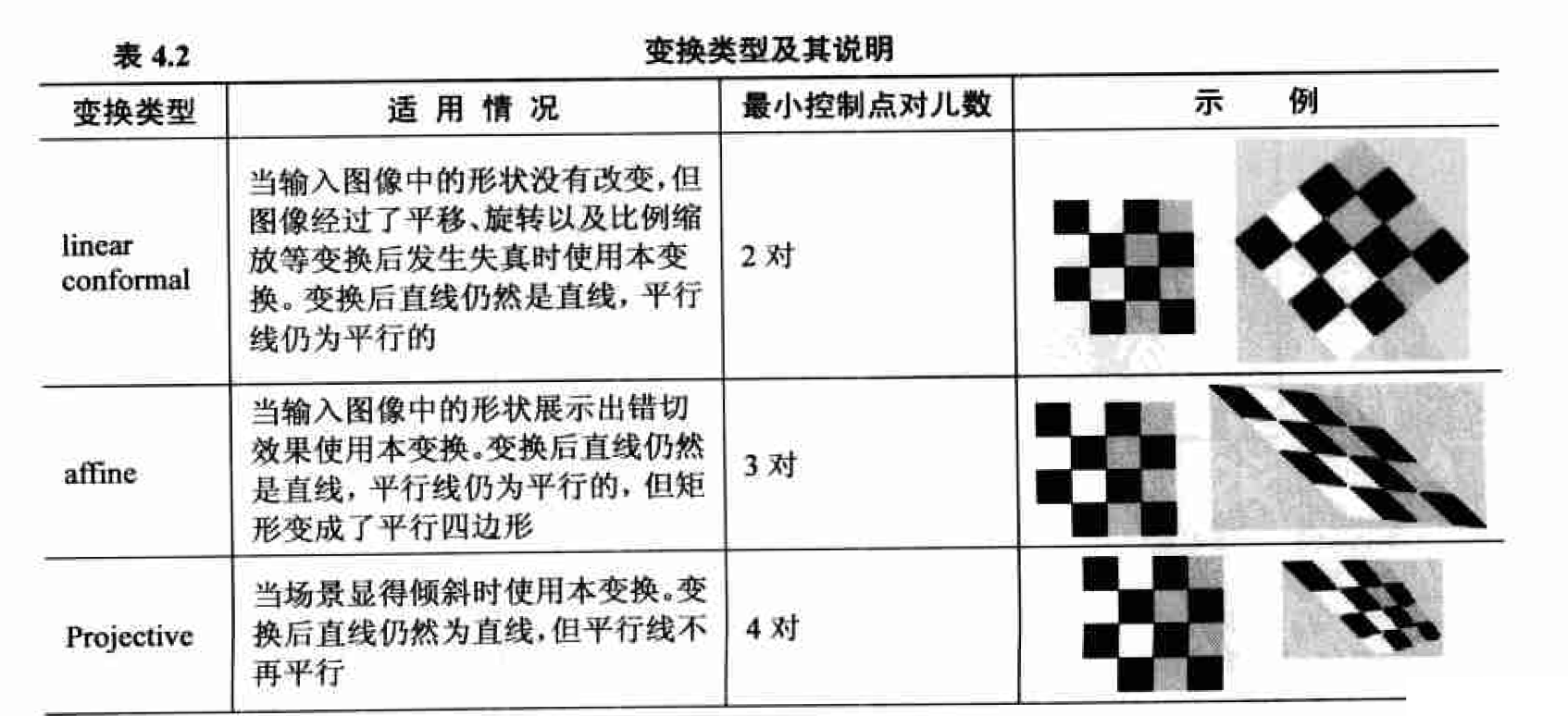# 图像的几何变换

Posted by jjx on December 21, 2016

• 图像的各种几何变换， 如平移、镜像和旋转等
• 插值算法
• 图像配准
• 本章的典型案例分析
• 基于直方图均衡化的图像灰度归一化
• 汽车牌照的投影失真校正

#### 解决几何变换的一般思路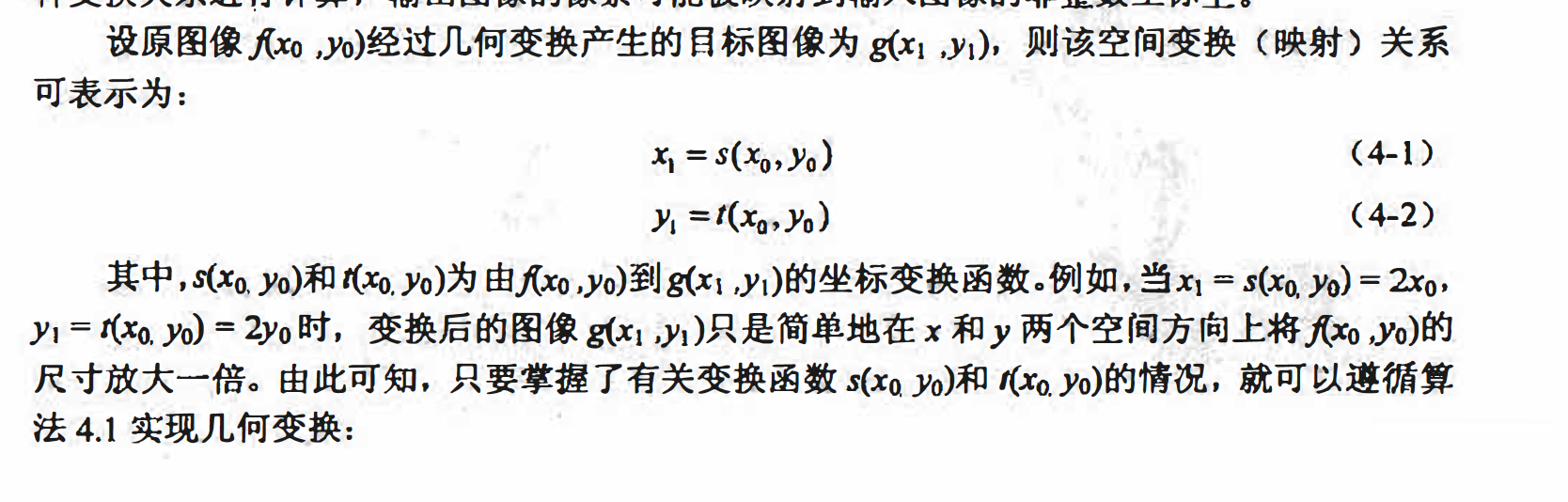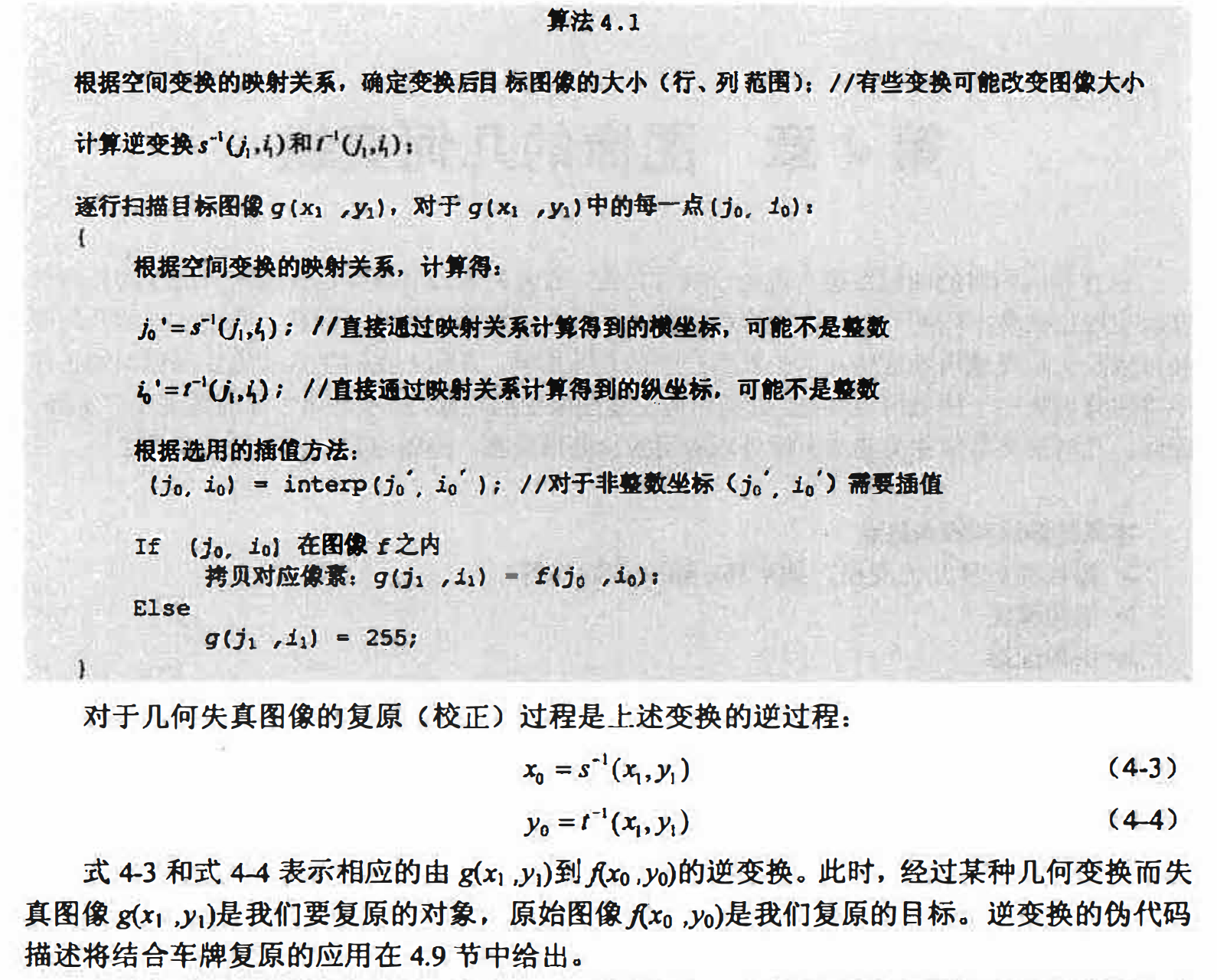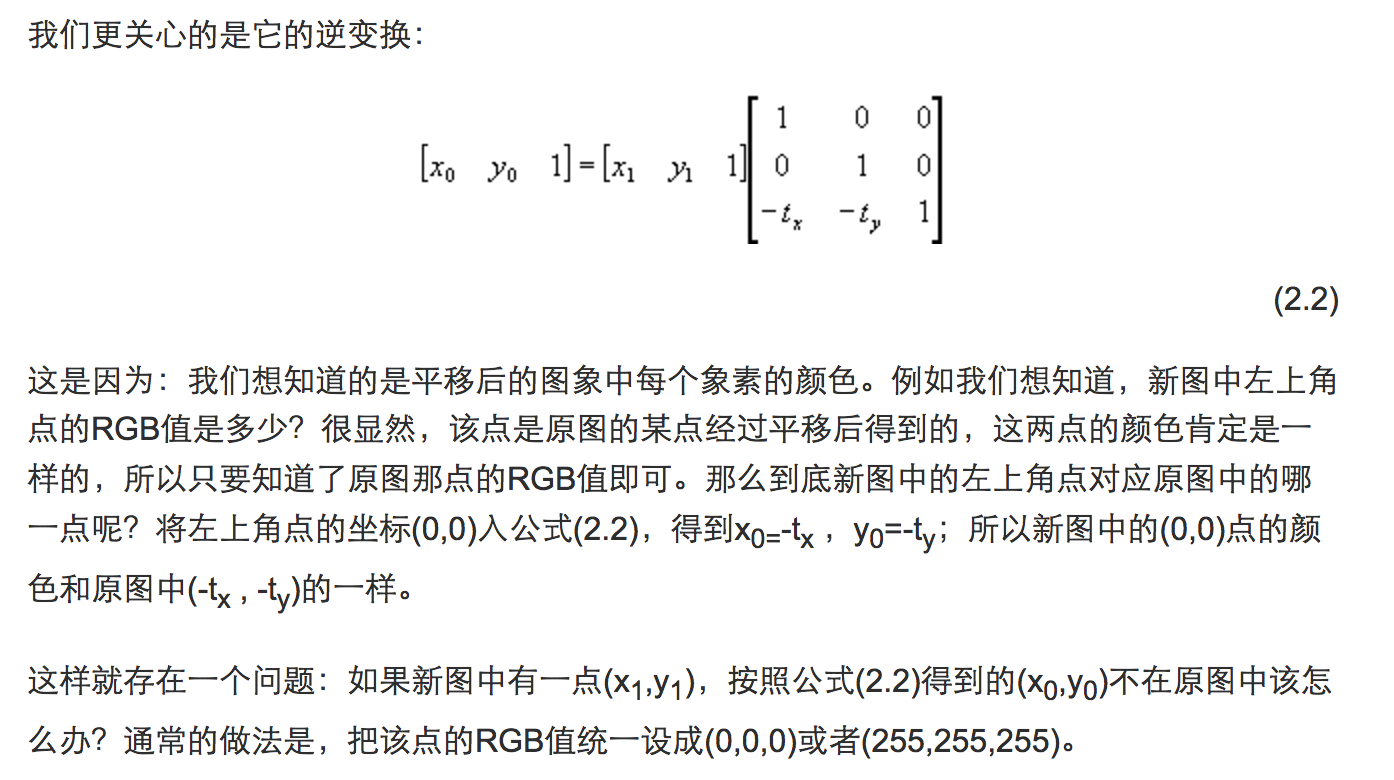#### 图像平移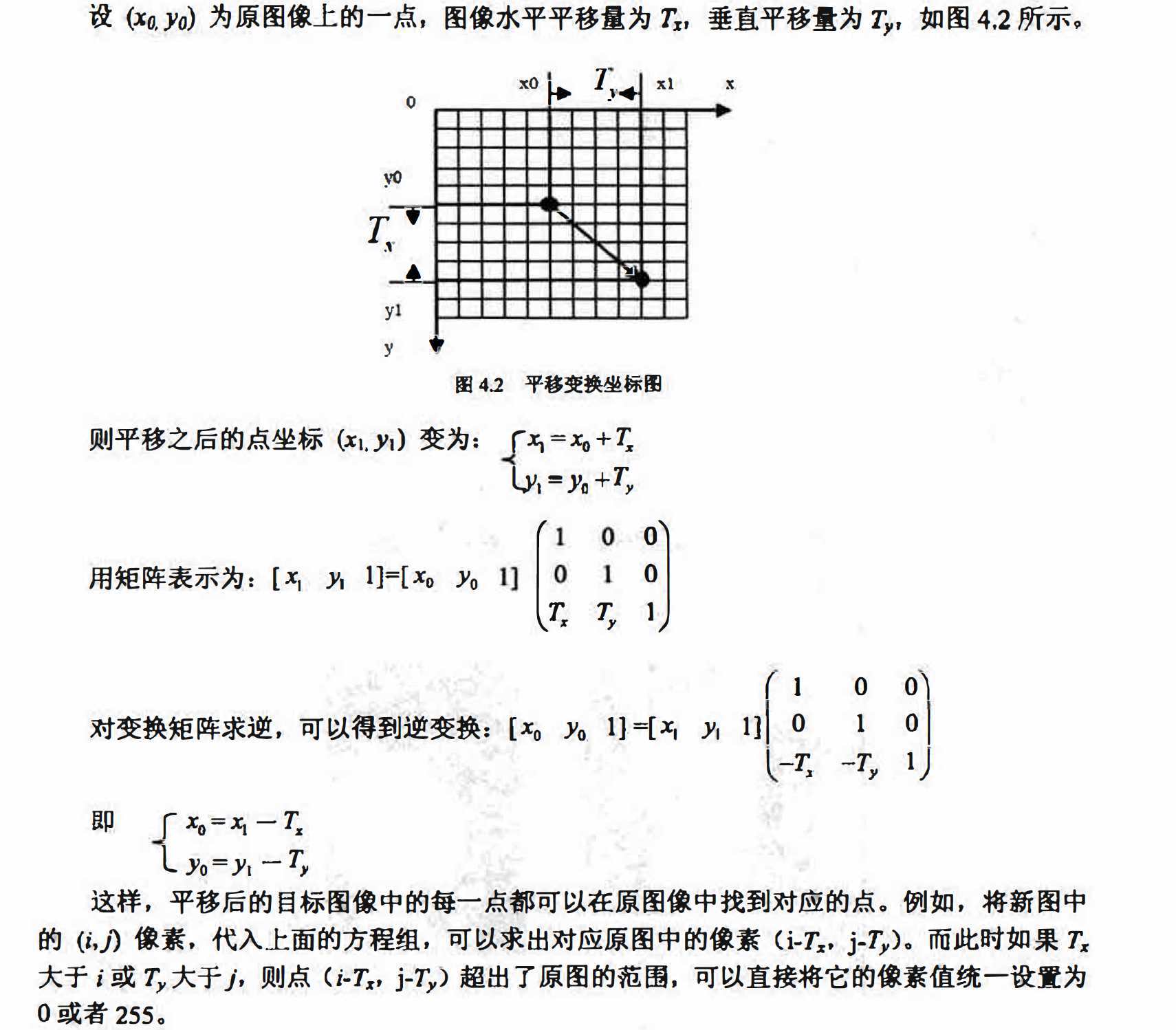init = imread('lena.bmp'); % 读取图像
[R, C] = size(init); % 获取图像大小
res = zeros(R, C); % 构造结果矩阵。每个像素点默认初始化为0（黑色）
delX = 50; % 平移量X
delY = 50; % 平移量Y
tras = [1 0 delX; 0 1 delY; 0 0 1]; % 平移的变换矩阵

for i = 1 : R
for j = 1 : C
temp = [i; j; 1];
temp = tras * temp; % 矩阵乘法
x = temp(1, 1);
y = temp(2, 1);
% 变换后的位置判断是否越界
if (x <= R) & (y <= C) & (x >= 1) & (y >= 1)
res(x, y) = init(i, j);
end
end
end;

figure;
subplot(1,2,1);
imshow(uint8(init)); title('原图像');
subplot(1,2,2);
imshow(uint8(res)); % 显示图像
title('平移后');#### 图像镜像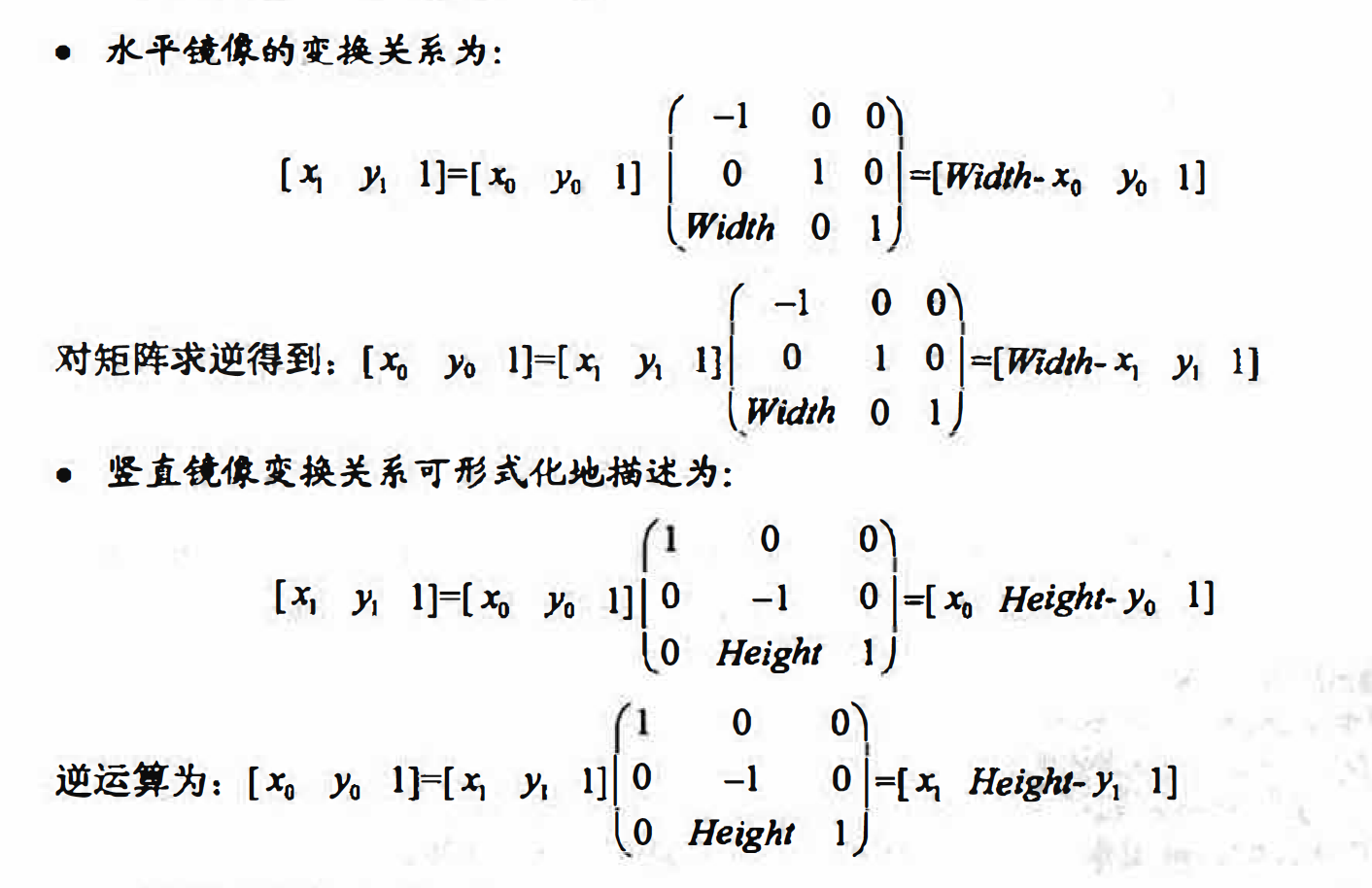matlab实现
imtransform函数用于完成一般的二维空间变换，形式如下:
B=imtransform(A,TFrom,method)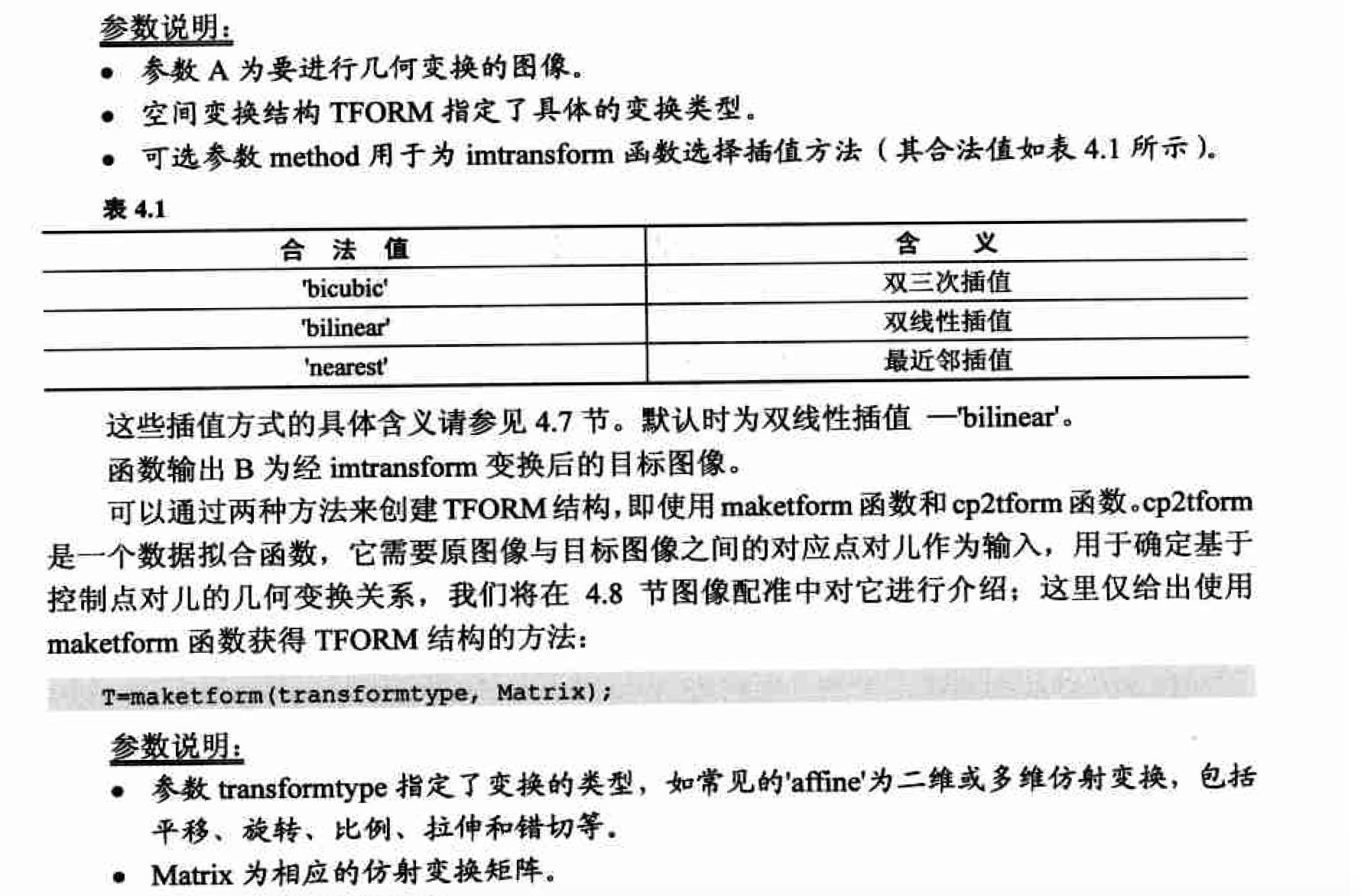A=imread('lena.bmp');
[height,width,dim]=size(A);
tform= maketform('affine',[-1 0 0;0 1 0;width 0 1]);

B = imtransform(A,tform,'nearest');
tform2=maketform('affine',[1 0 0;0 -1 0;0 height 1]);
C = imtransform(A,tform2,'nearest');

subplot(1,3,1),imshow(A);
title('原图像');
subplot(1,3,2),imshow(B);
title('水平镜像');
subplot(1,3,3),imshow(C);
title('竖直镜像');#### 图像转置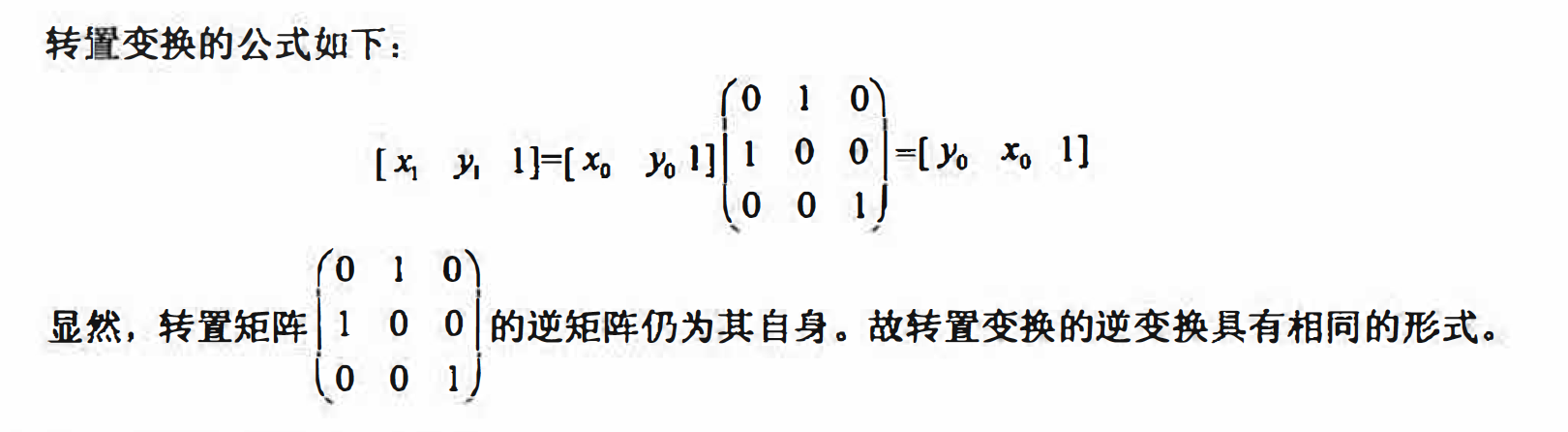matlab实现

A = imread('lena.bmp');
tform = maketform('affine',[0 1 0;1 0 0;0 0 1]);
B = imtransform(A,tform,'nearest');

subplot(1,2,1),imshow(A);
title('原图像');
subplot(1,2,2),imshow(B);
title('图像转置');#### 图像缩放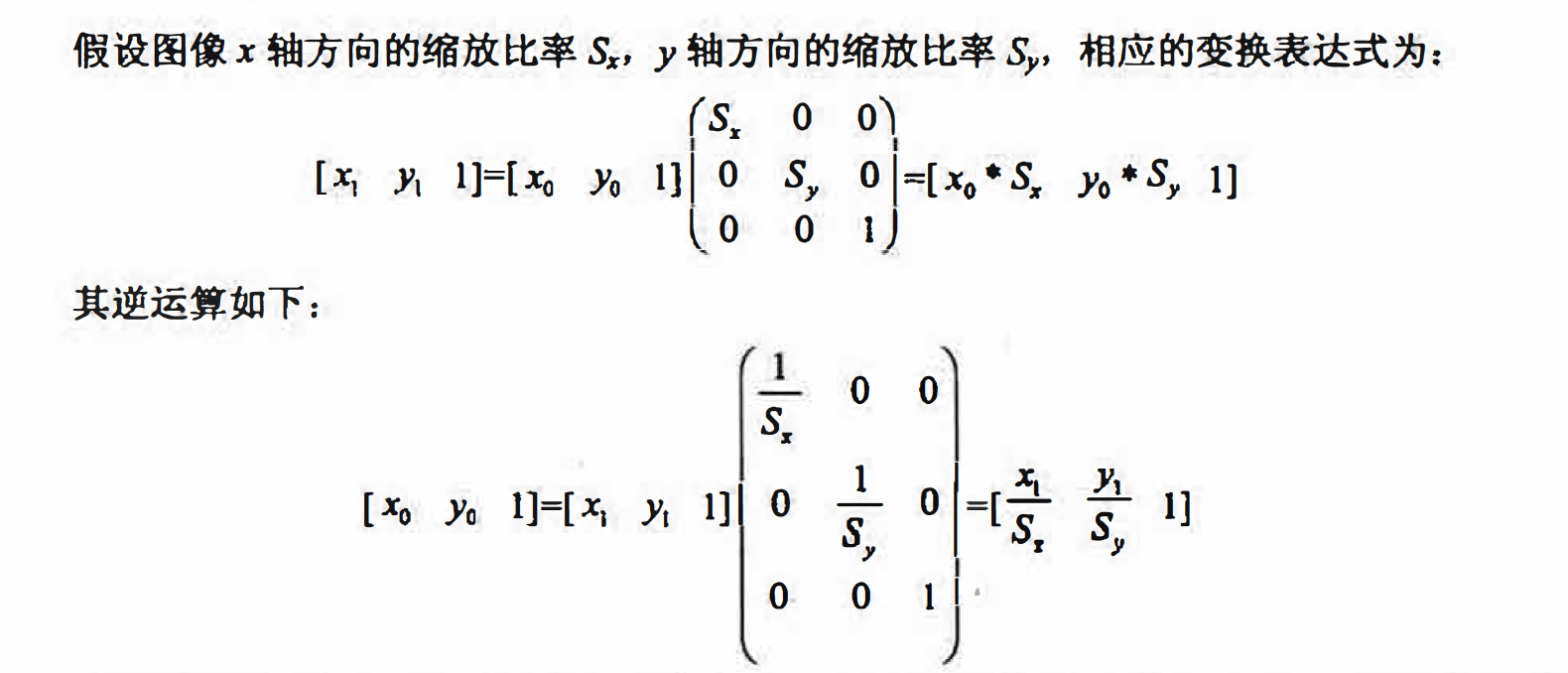matlab实现

B = imresize(A,Scale,method);

a为缩放的原始图像
Scale为统一缩放比例
method用于指定插值方法，其合法取值同imtransform

A = imread('lena.bmp');
B = imresize(A,1.2,'nearest');

figure,imshow(A);title('原图像');
figure,imshow(B);title('图像缩放');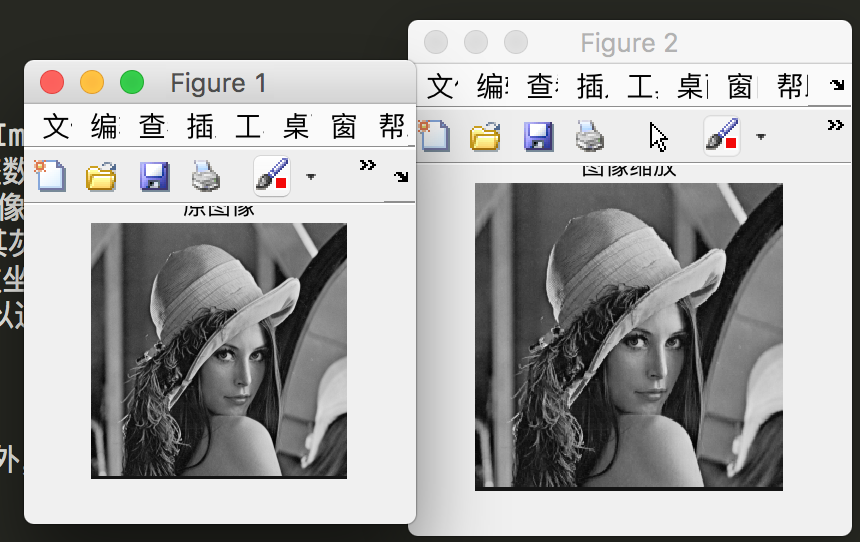#### 图像旋转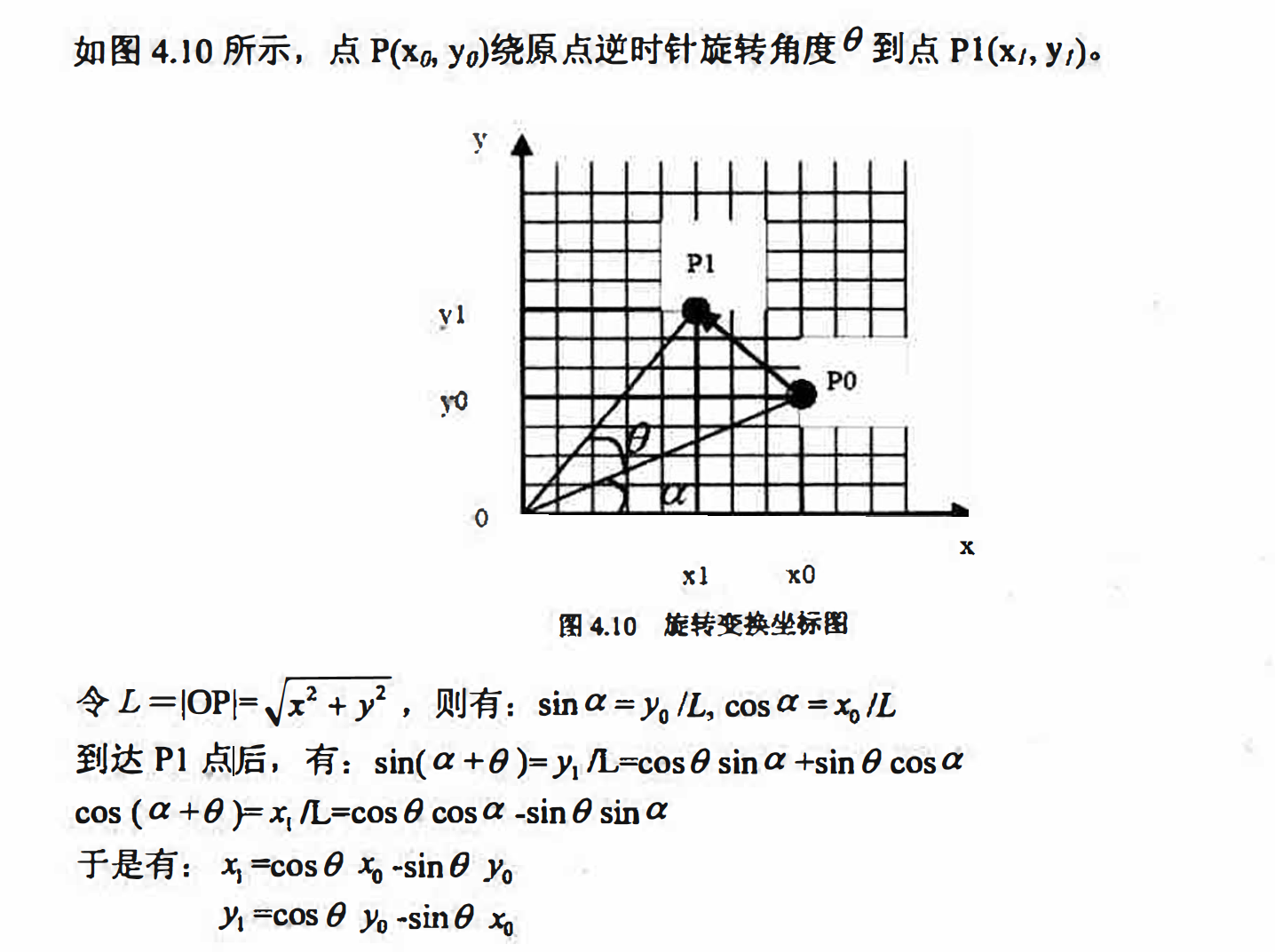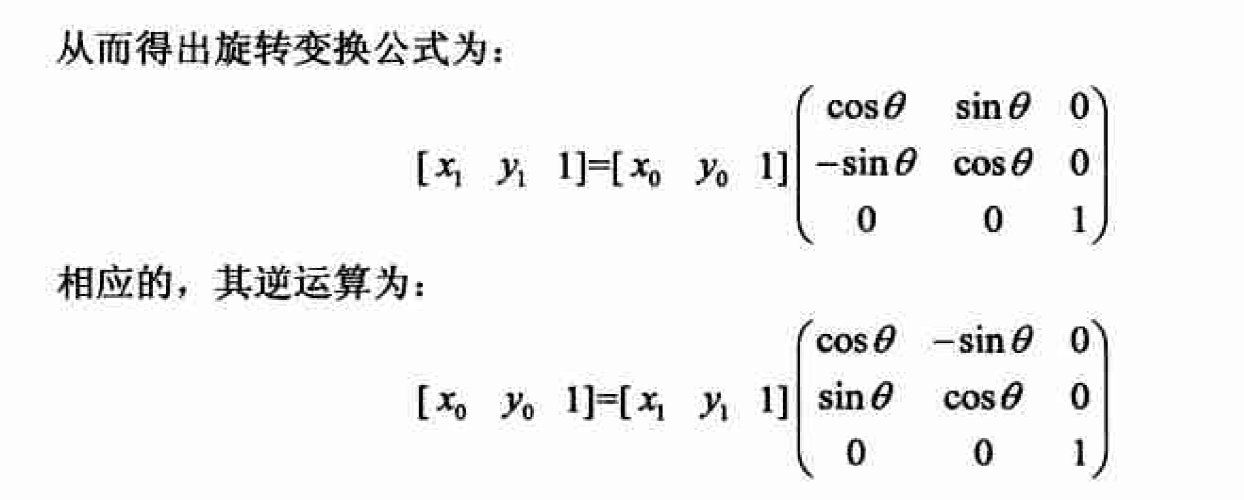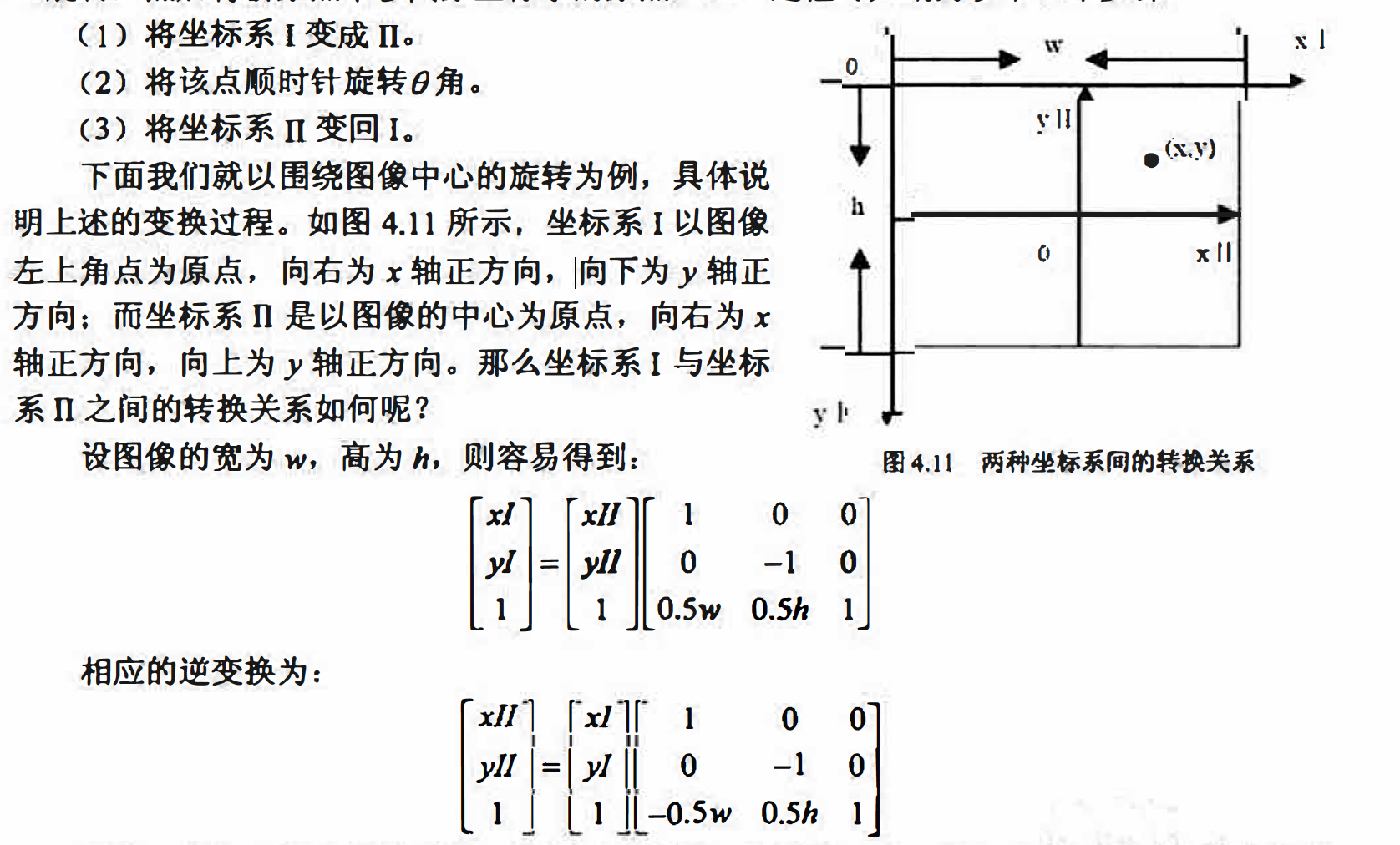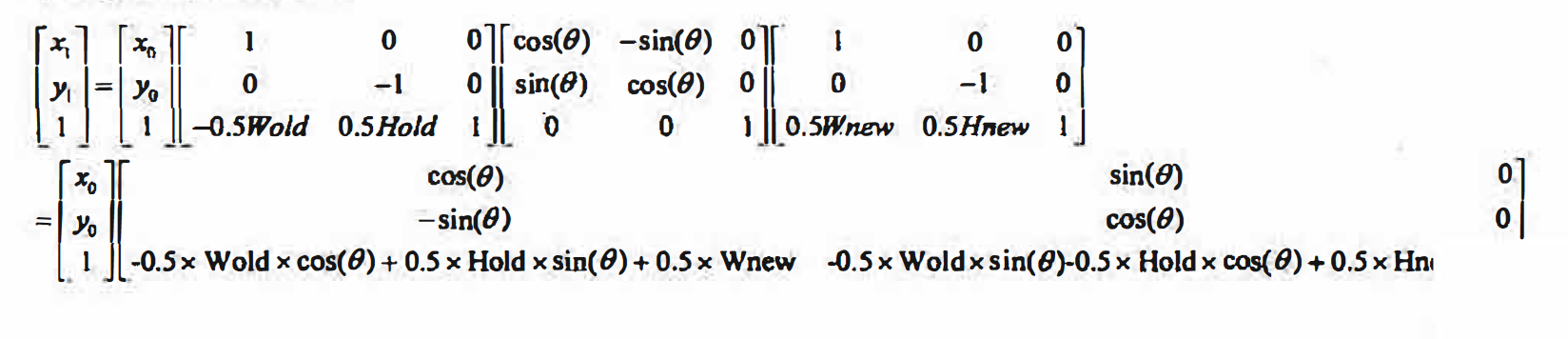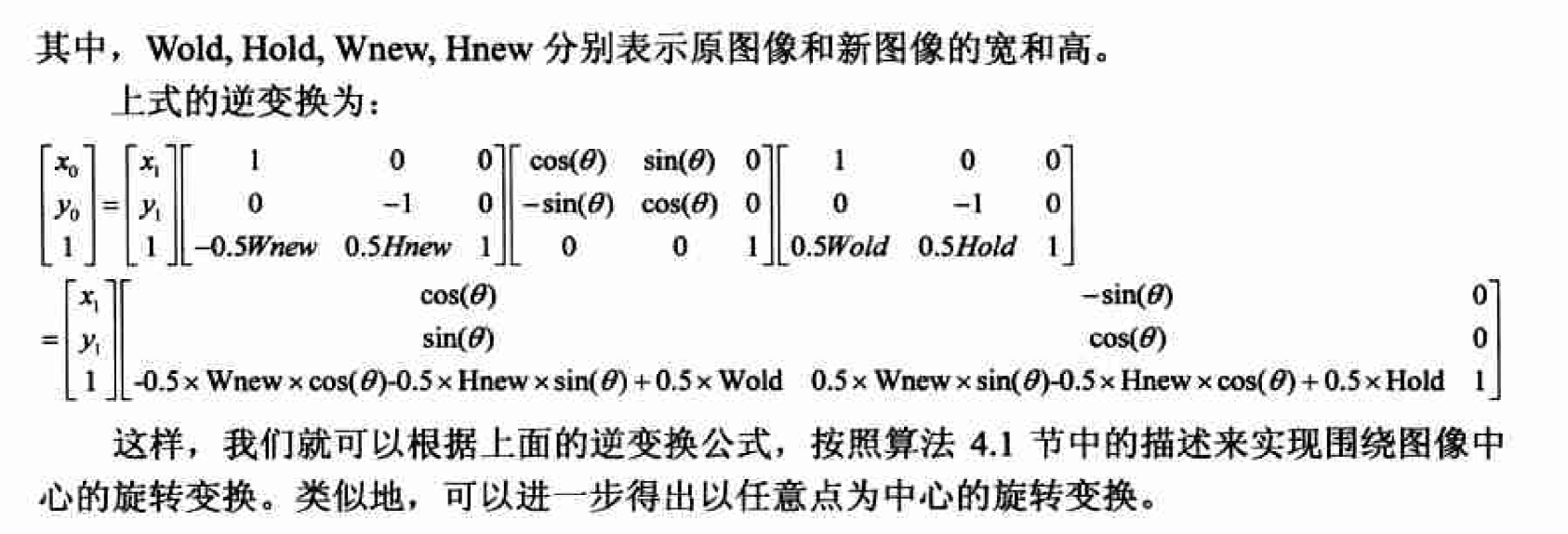matlab实现

B=imrotate(A,angle,method,’crop’)

• A是要旋转的图像．
• angle 为旋转角度，单位为度，如果为其指定一个正值，则imrotate 函数接逆时针方 向旋转圈像． · 可选参数method为imrotate 函数指定插佳方法． • ‘crop＇选项会裁剪旋转后增大的图像，使得到的圈像和原图大小一致．
A = imread('lena.bmp');
B = imrotate(A,30,'nearest','crop');
subplot(1,2,1),imshow(A);
title('原图像');
subplot(1,2,2),imshow(B);
title('逆时针旋转30度');### 插值算法

#### 最近邻插值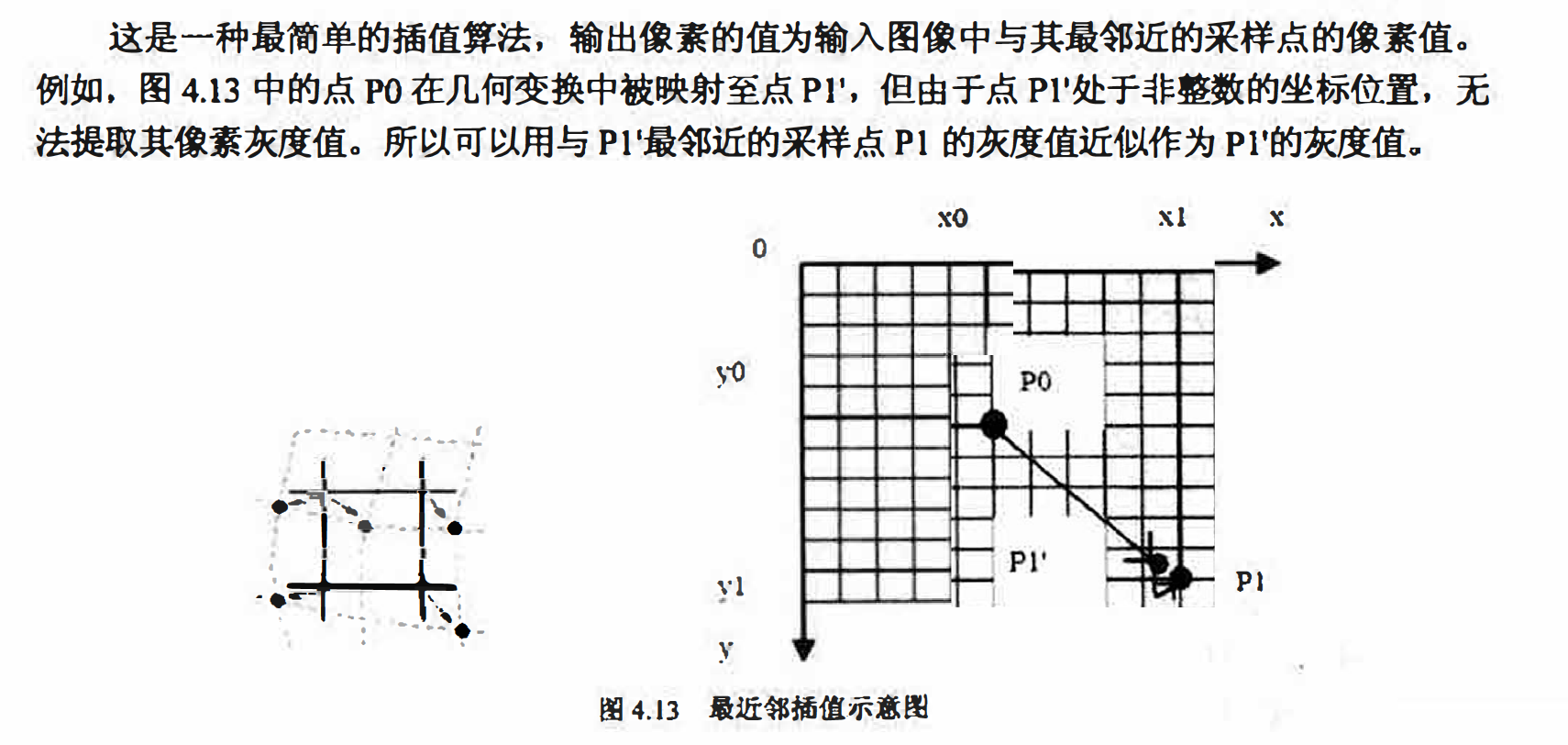f(x,y) = g(round(x)， round (y) );

#### 双线性插值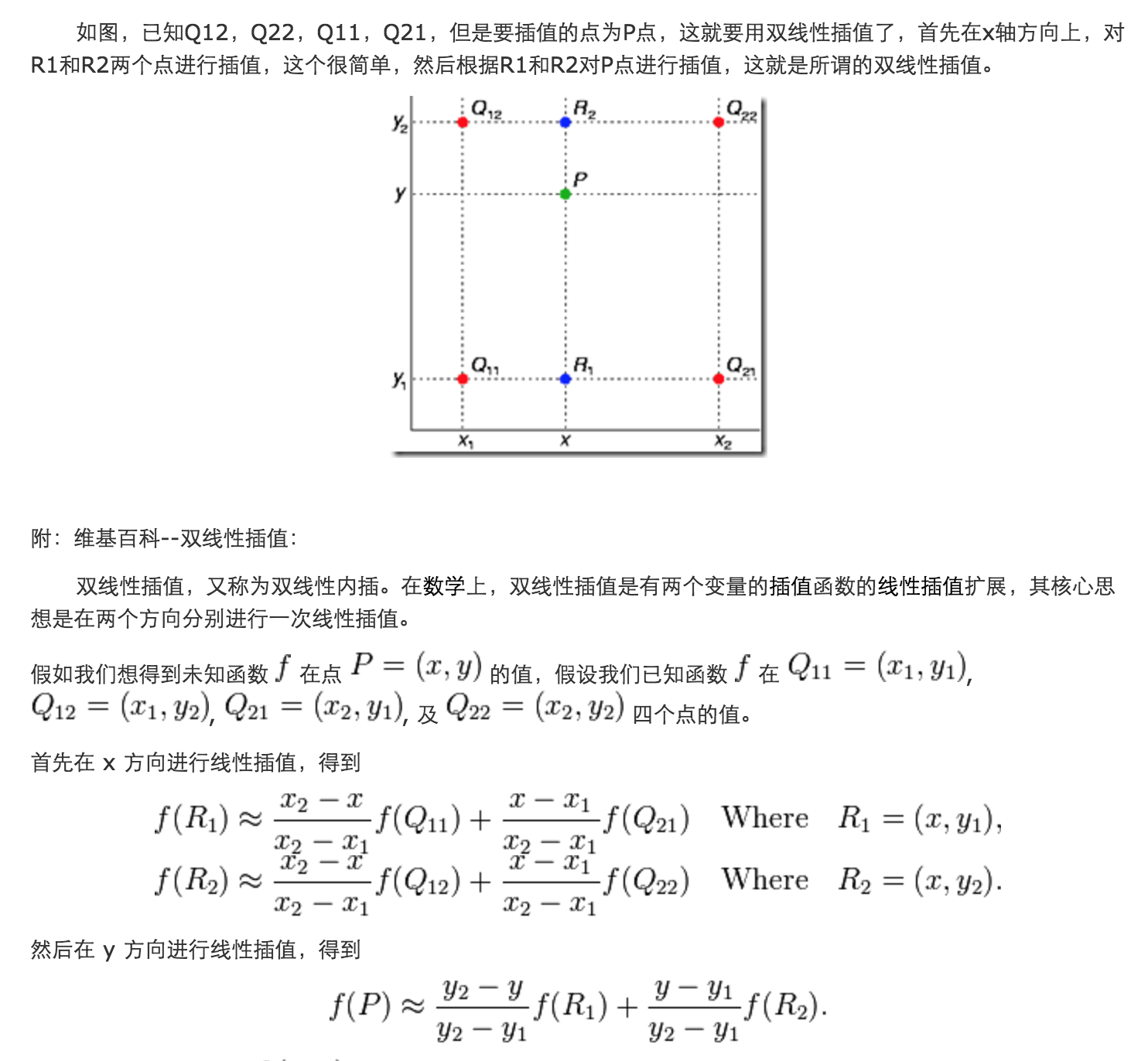#### 高阶插值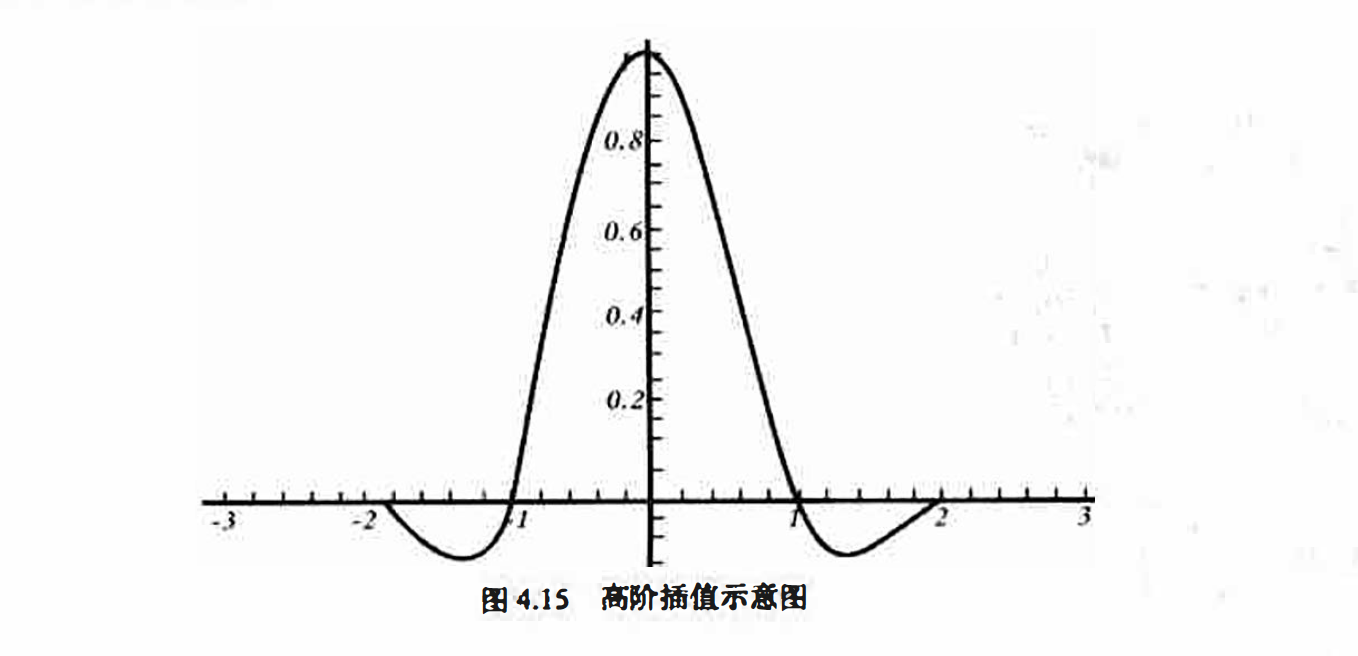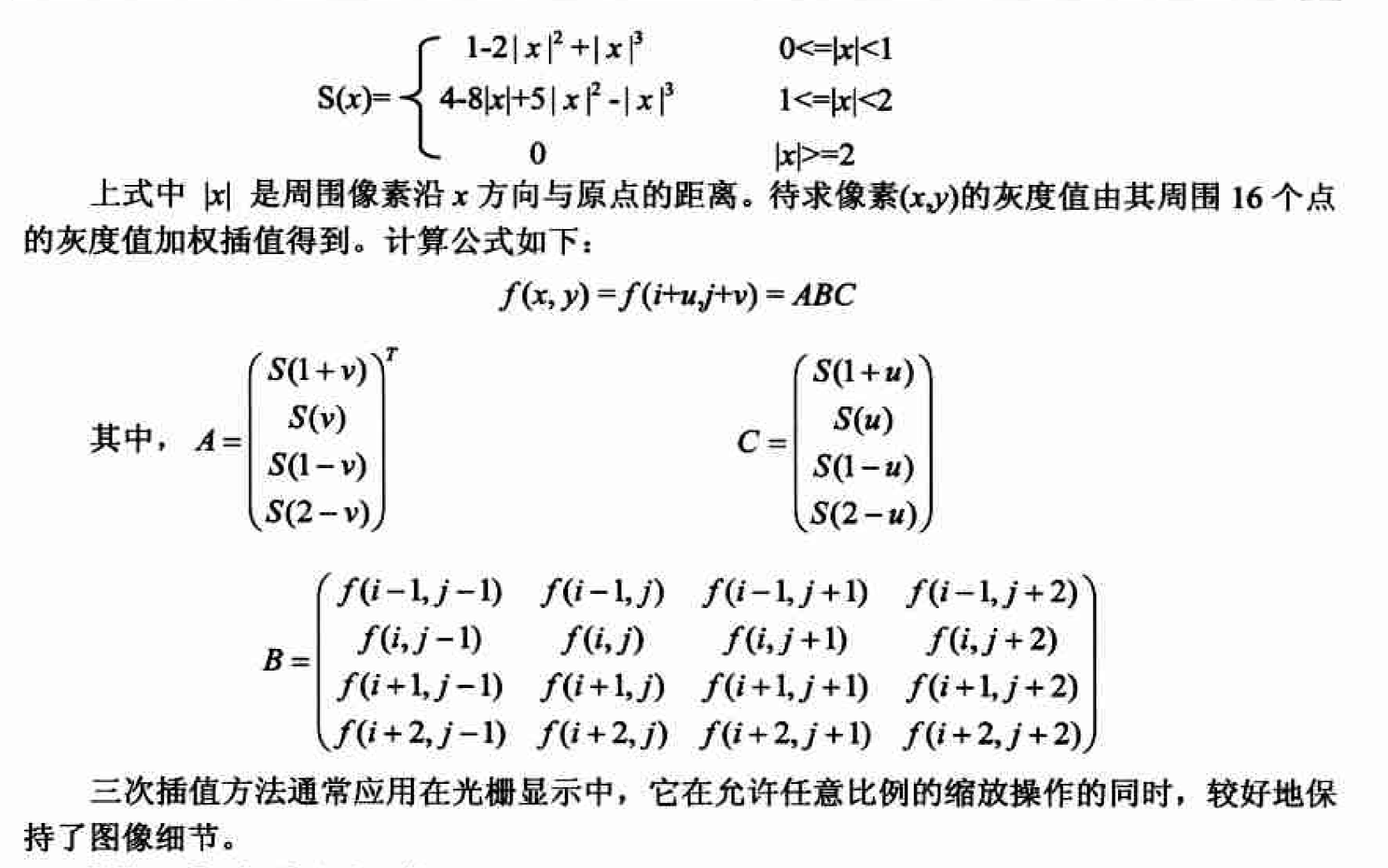A = imread('rectangle.bmp');
B = imrotate(A,30,'nearest');
C = imrotate(A,30,'bilinear');
D = imrotate(A,30,'bicubic');

subplot(2,2,1),imshow(A);
title('原图像');
subplot(2,2,2),imshow(B);
title('最近邻插值');
subplot(2,2,3),imshow(C);
title('双线性插值');
subplot(2,2,4),imshow(D);
title('双三次插值');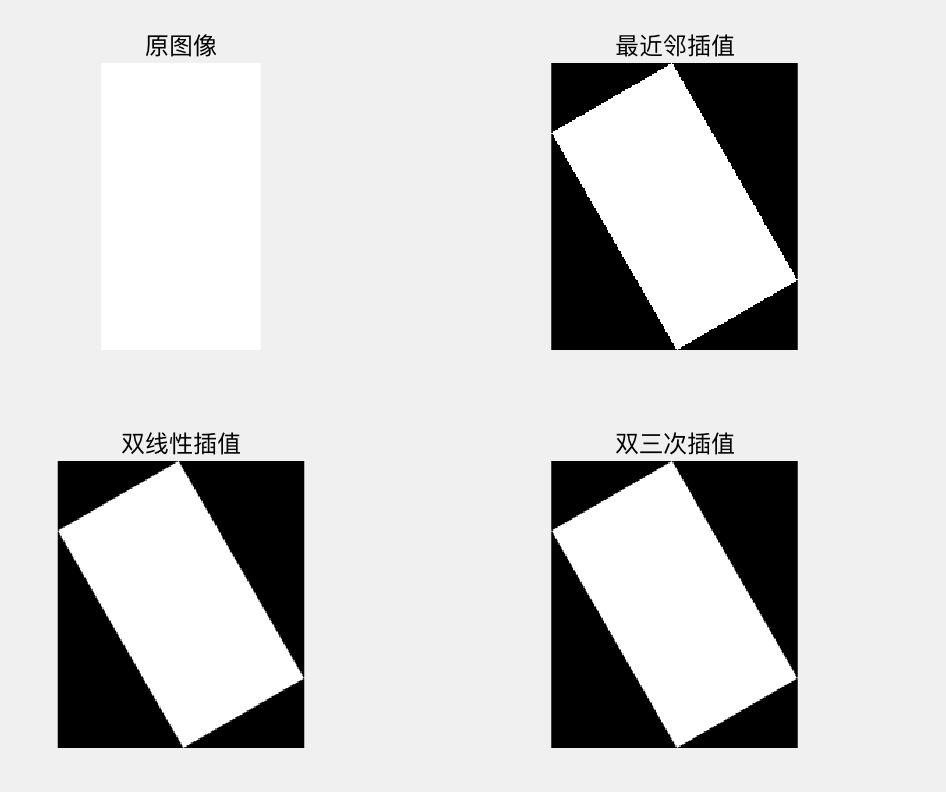#### 图像配准

Iin = imread('lena2.bmp');